# Rotation - using logic

## Determining new coordinates using logic

You can determine the new coordinates of an object using:

Rotation & the "L" shape

It works by joining the origin with the point to be rotated with an "L" shape.

Example 1

Rotate the following object clockwise 90° from the centre (1,3).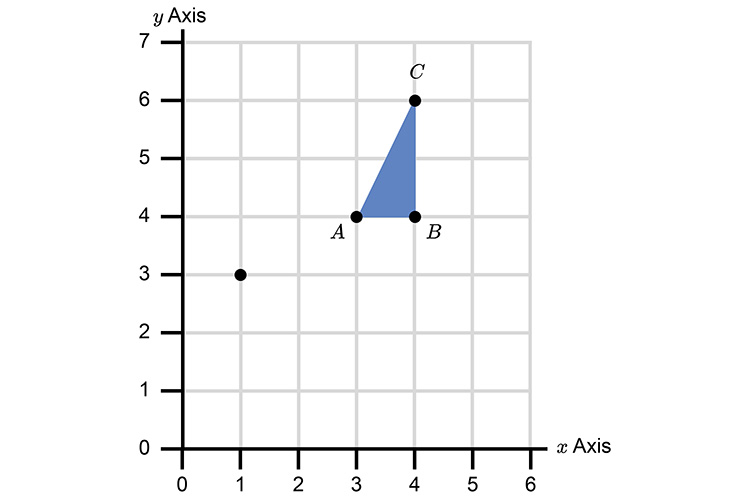Join the origin to A with an L.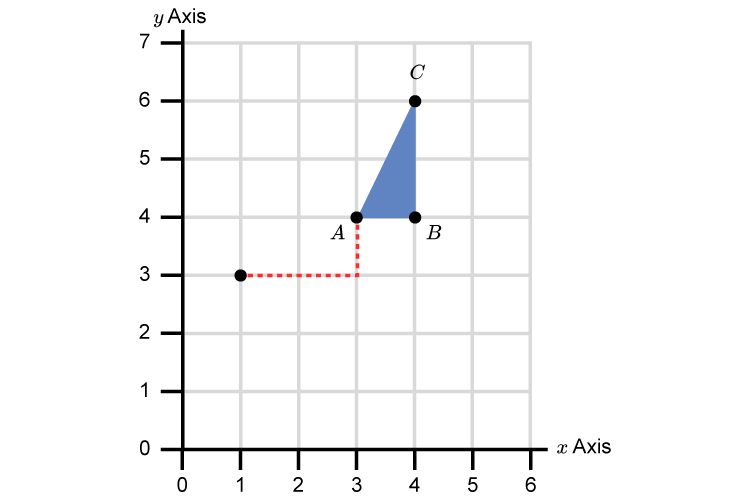Now we are only dealing with right angles which makes this very easy when we want to turn a point through 90° clockwise.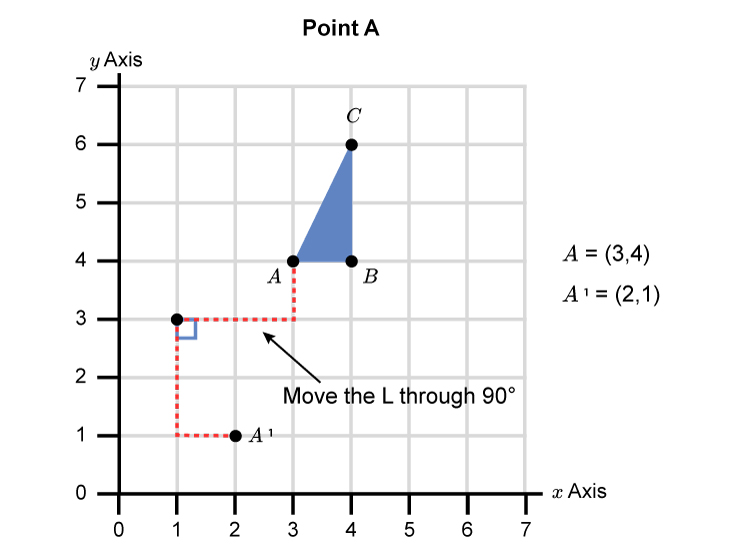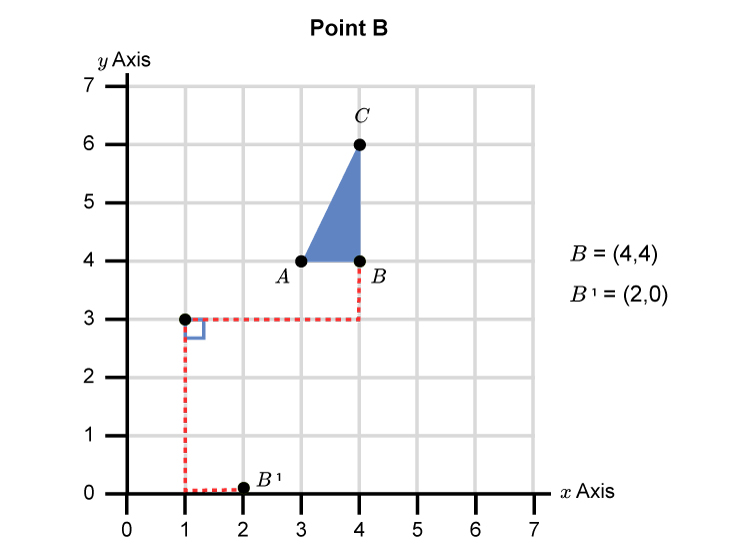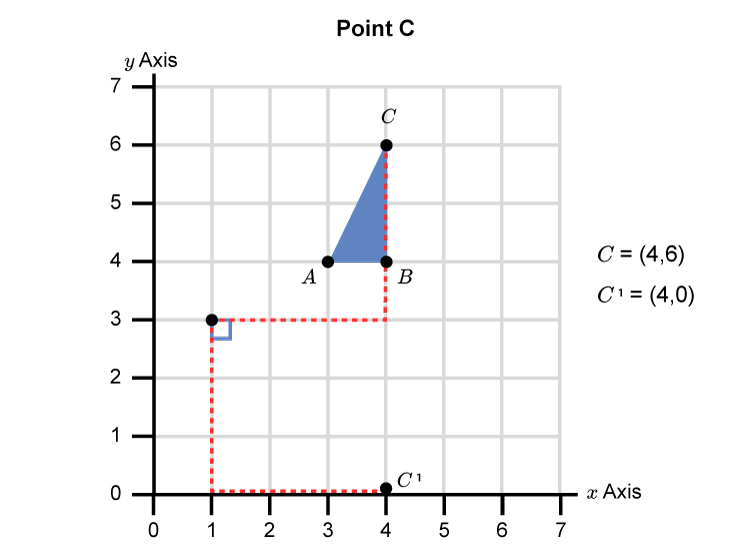This gives a final graph as: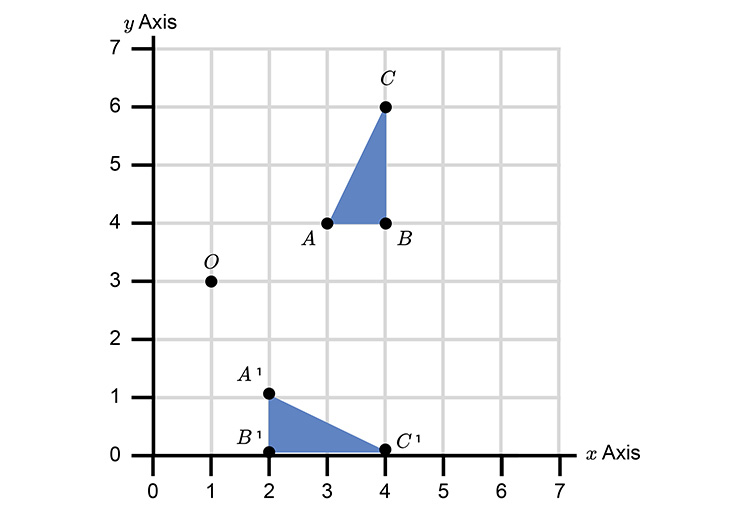A, B, C has been rotated clockwise 90° from centre (1,3) to give the new triangle A^1, B^1, C^1.

Example 2

Rotate the following triangle 180° about the origin (0,0).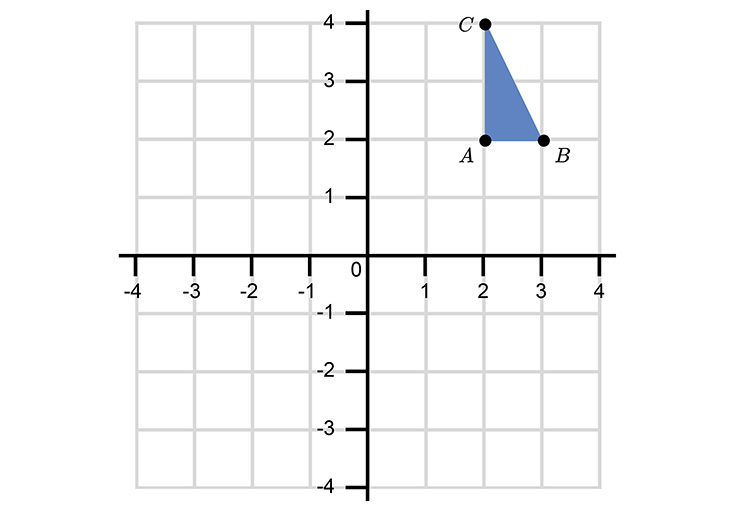Rotate point A  180° using the L shape.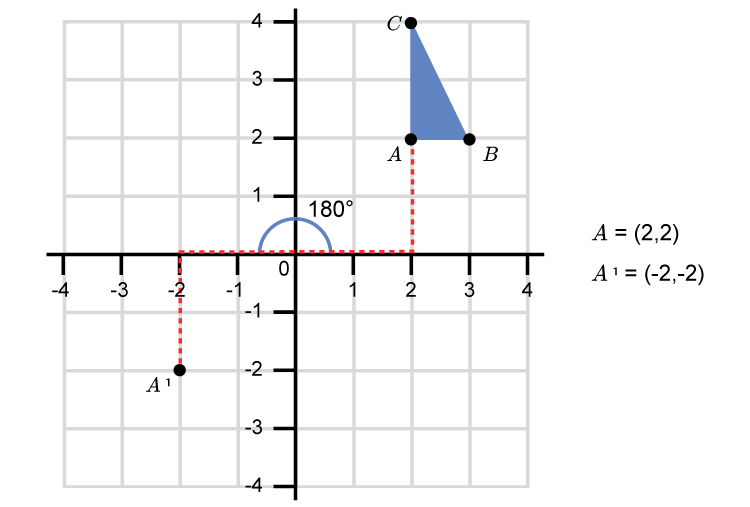Rotate point B  180° using the L shape.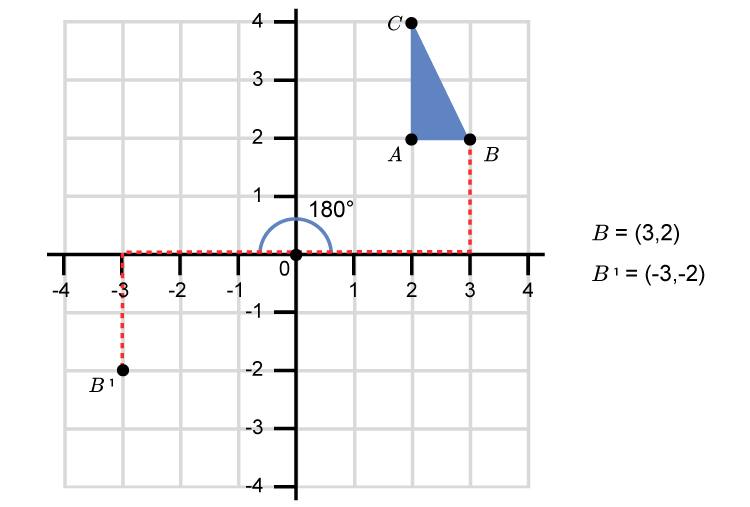Rotate point C  180° using the L shape.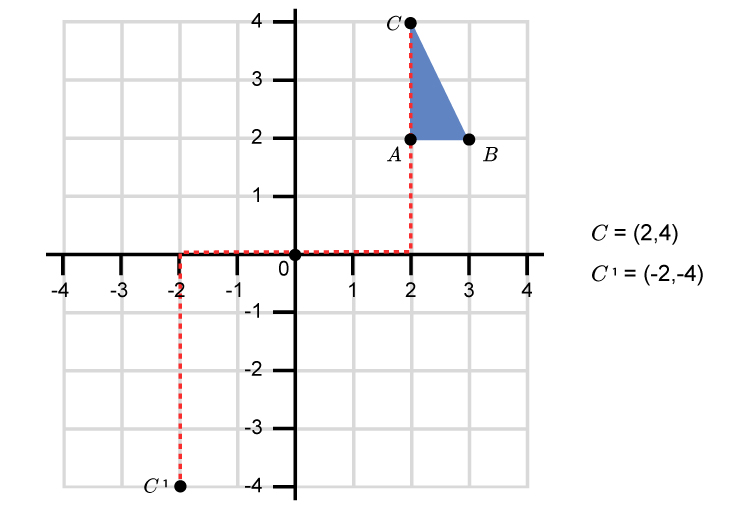This gives a final graph as: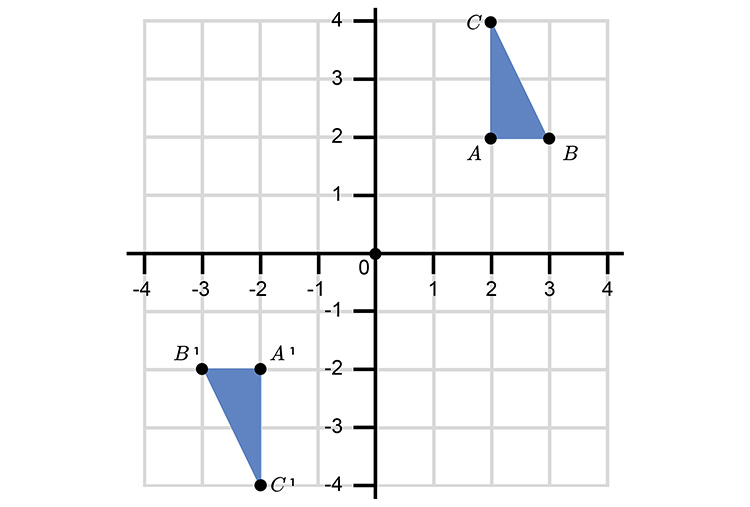A, B, C has been rotated 180° from the centre (0,0) to give the new triangle A^1, B^1, C^1.

Example 3

Rotate the following shape 90° anticlockwise about grid point (1,1).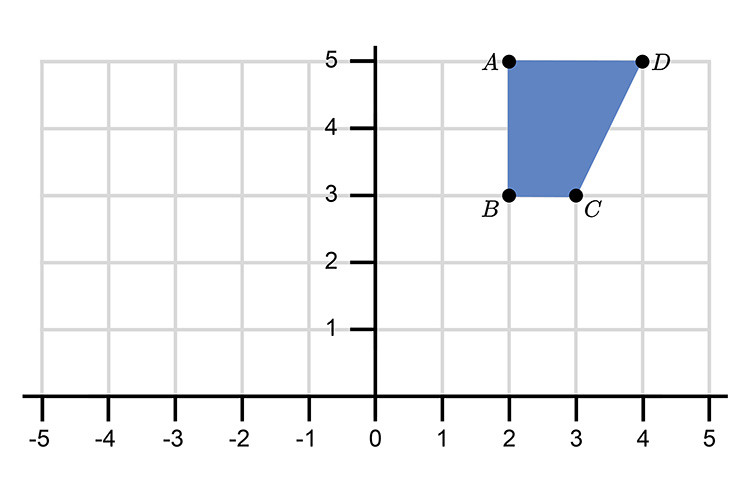Rotate point A  90° using the L shape.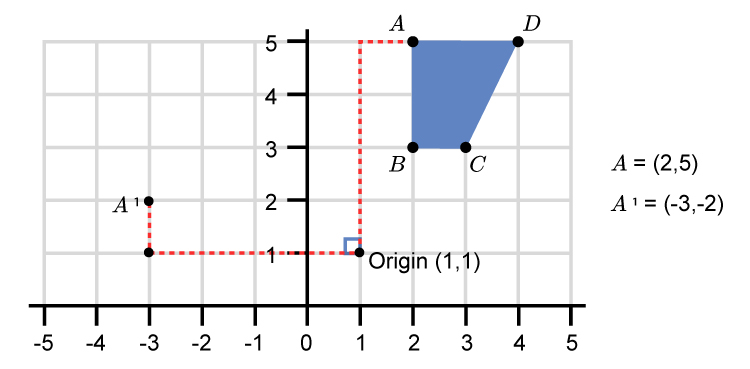Rotate point B  90° using the L shape.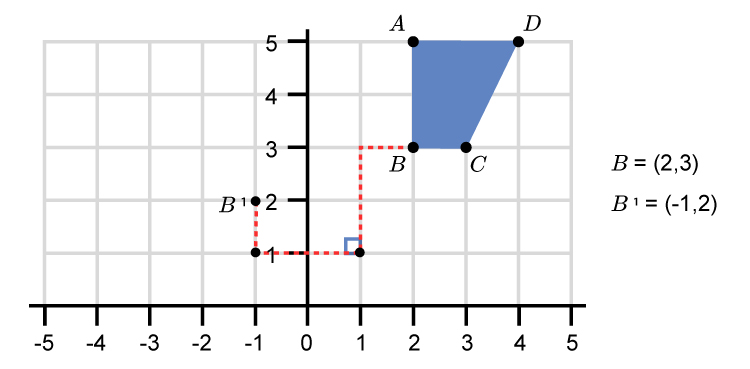Rotate point C  90° using the L shape.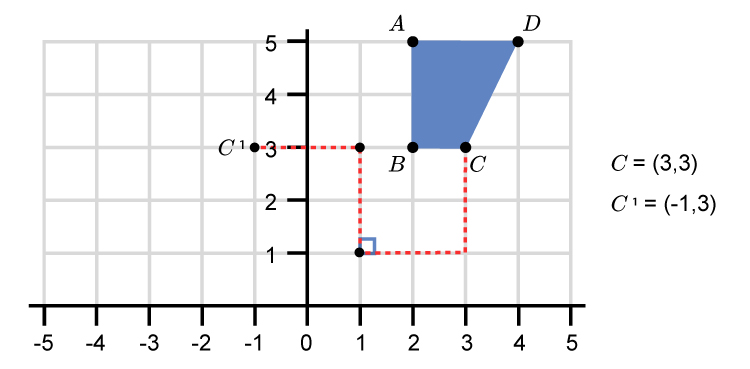Rotate point D  90° using the L shape.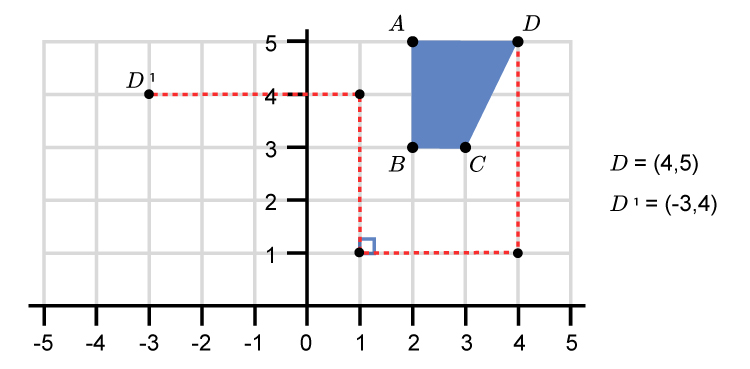This gives a final graph as: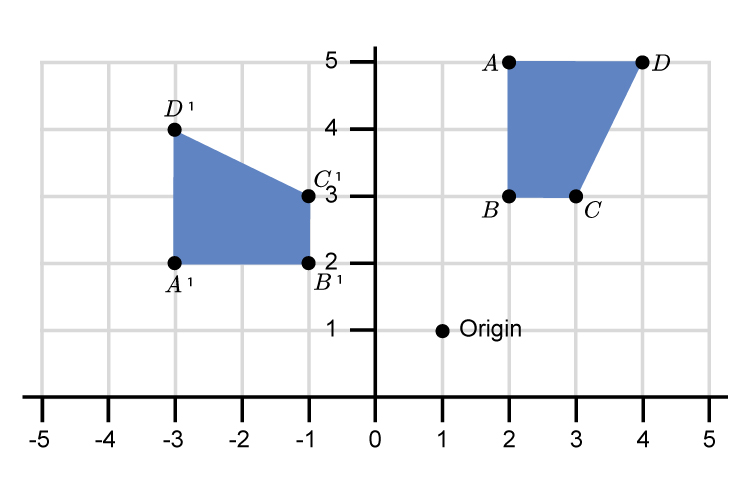A,B,C,D has been rotated 90° anti clockwise about centre (1,1) to give A^1,B^1,C^1,D^1.